# HBSE 10th Class Maths Important Questions Chapter 4 Quadratic Equations

Haryana State Board HBSE 10th Class Maths Important Questions Chapter 4 Quadratic Equations Important Questions and Answers.

## Haryana Board 10th Class Maths Important Questions Chapter 4 Quadratic Equations

Question 1.
If x = 3 is one root of quadratic equation x2 – 2kx – 6 = 0, then find the value of k.
Solution :
Since, x = 3 is one root of the quadratic equation x2 – 2kx – 6 = 0. So, we put x = 3 in the given equation, we get
(3)2 – 2k × 3 – 6 = 0
⇒ 9 – 6k – 6 = 0
⇒ – 6k + 3 = 0
⇒ k = $$\frac {3}{6}$$
⇒ k = $$\frac {1}{2}$$Question 2.
If one root of the quadratic equation 6x2 – x – k = 0 is $$\frac {2}{3}$$ then find the value of k.
Solution :
Since, $$\frac {2}{3}$$ is one root of the quadratic equation 6x2 – x – k = 0.
So, we put x = $$\frac {2}{3}$$ in the given equation, we get
6 × ($$\frac {2}{3}$$)2 – $$\frac {2}{3}$$ – k = 0
⇒ 6 × $$\frac{4}{9}-\frac{2}{3}$$ – k = 0
⇒ $$\frac{8}{3}-\frac{2}{3}$$ – k = 0
⇒ $$\frac {6}{3}$$ – k = 0
⇒ 2 – k = 0
⇒ k = 2

Question 3.
Find the possible root of $$\sqrt{3 x^2+6}$$ = 9.
Solution :
The given equation is
$$\sqrt{3 x^2+6}$$ = 9
Squaring on both sides, we get
3x2 + 6 = 81
⇒ 3x2 = 81 – 6
⇒ 3x2 = 75
⇒ x2 = $$\frac {75}{3}$$
⇒ x2 = 25
Taking square root both sides, we get
$$\sqrt{x^2}$$ = $$\sqrt{25}$$
x = ± 5Question 4.
Solve : $$\frac{1}{a+b+x}=\frac{1}{a}+\frac{1}{b}=\frac{1}{x}$$ where a + b ≠ 0.
Solution :
The given equation is :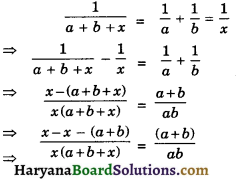⇒ – (a + b) × ab = (a + b)x (a + b + x)
⇒ (a + b)x (a + b + x) + ab (a + b) = 0
⇒ (a + b) [x(a + b + x) + ab] = 0
⇒ x (a + b + x) + ab = 0
⇒ ax + bx + x2 + ab = 0
⇒ x2 + ax + bx + ab = 0
⇒ x(x + a) + b (x + a) = 0
⇒ (x + a) (x + b) = 0
⇒ (x + a) = 0 and (x + b) = 0
⇒ x = – a and x = – b

Question 5.
A train travels 300 km at a uniform speed. If the speed had been 10 km/hr more, it would have 1 hr less for same journey. Find the speed of train.
Solution :
Let the speed of train be x km/hr
Distance = 300 km (given)
Time taken by train = $$\frac {300}{x}$$hrs.
[∵ Time = Distance / Speed]
If speed had been 10 km/hr more then new speed = (x + 10) km/hr.
Time taken = $$\frac{360}{x+10}$$ hrs.
According to question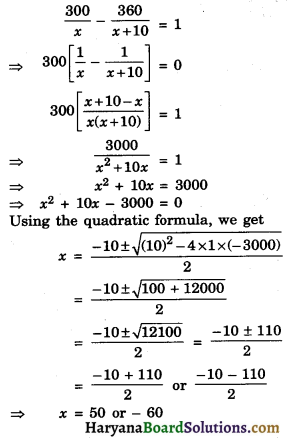Question 6.
The diagonal of a rectangular field is 25 metres more than the shorter side. If longer side is 23 metres more than the shorter side, find the sides of the field.
Solution :
Let the shorter side be x m. Then diagonal = (x + 25)m and longer side = (x + 23)m.
We known that each angle of a rectangle is 90°. In a right ΔABC, we have (x + 25)2 = (x + 23)2 + x2.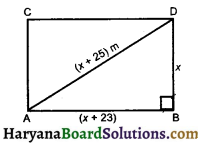⇒ x2 + 50x + 625 = x2 + 46x + 529 + x2
⇒ 2x2 + 46x + 529 – x2 – 50x – 625 = 0
⇒ x2 – 4x – 96 = 0
⇒ x2 – (12 – 8)x – 96 = 0
⇒ x2 – 12x + 8x – 96 = 0
⇒ (x2 – 12x) + (8x – 96) = 0
⇒ x(x – 12) + 8 (x – 12) = 0
⇒ (x – 12) (x + 8) = 0
⇒ x – 12 = 0 and x + 8 = 0
⇒ x = 12 and x = – 8 (Reject)
Hence, longer side 12 + 23 i.e., 35 m and shorter side = 12 m.Question 7.
In a flight of 600 km, an discraft was slowed down due to bad weather. The average speed of the strip was reduced by 200 km/hr and time of flight increased by 30 minutes. Find the duration of flight.
Solution :
Distance = 600 km.
Let the speed of discraft be x km/hr. Time taken by discraft (T1) = $$\frac {600}{x}$$ hrs.
It speed of is craft was slow down by 200 km/ hr then new speed = (x – 200) km/hr.
Time taken by discraft (T2) = $$\frac{600}{x-200}$$ hrs
According to question,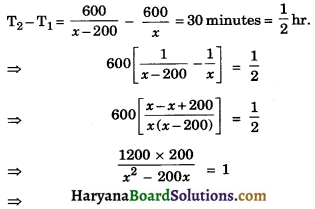⇒ 2,40,000 = x2 – 200x
⇒ x2 – 200x – 2,40,000 = 0
⇒ x2 – (600 – 400)x – 2,40,000 = 0
⇒ x2 – 600x + 400x – 2,40,000 = 0
⇒ x(x – 600) + 400(x – 600) = 0
⇒ (x – 600) (x + 400) = 0
⇒ x – 600 = 0 and x + 400 = 0
⇒ x = 600 and x = – 400 (Reject)
So, speed of discraft = 600 km/hr and duration of flight = $$\frac{600}{600-200}$$ hrs
= $$\frac{600}{400}=\frac{3}{2}$$ = 1$$\frac {1}{2}$$hrs.

Question 8.
The speed of a boat in still water is 18 km/hr. It takes $$\frac {1}{2}$$ an hours extra in going 12 km upstream instread of going the same distance down stream. Find the speed of the stream.
Solution :
Let the speed of stream be x km/hr. Speed of boat in still water = 18 km/hr (given)
Speed of boat in down stream = (18 + x) km/hr
Time taken in going down stream (T1) = $$\frac{12}{(18+x)}$$ hrs
Speed of boat in upstream (18 – x) km/hr.
Time taken in going upstream (T2) = $$\frac{12}{(18-x)}$$ hrs
According to question,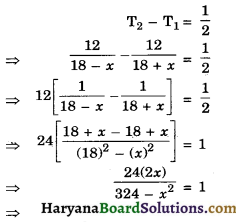⇒ 48x = – x2 + 324
⇒ x2 + 48x – 324 = 0
⇒ x2 + (54 – 6)x – 324 = 0
⇒ x2 + 54x – 6x – 324 = 0
⇒ x(x + 54) – 6(x + 54) = 0
⇒ (x + 54) (x – 6) = 0
⇒ x + 54 = 0 and x – 6 = 0
⇒ x = – 54 (Reject) and x = 6
Hence, speed of stream = 6 km/hr.Question 9.
I can take 12 hours to fill a swimming pool using two pipes. If the pipe of larger diameter is used for 4 hours and the pipe of smaller diameter for 9 hours, only half of the pool can be filled. How long would it take for each pipe to fill the pool separately ?
Solution :
Let the time taken by larger pipe be x hours and time taken by smaller pipe be y hours
The portion of tank filled by larger pipe in 1 hour = $$\frac {1}{x}$$part
The portion of tank filled by smaller pipe in 1 hour = $$\frac {1}{y}$$part
Two pipe can fill the tank in 12 hours.
∴ The portion of tank filled by both pipe in 1 hr = $$\frac {1}{12}$$part
According to condition 1st
$$\frac{1}{x}+\frac{1}{y}=\frac{1}{12}$$ ……………(1)
If larger pipe used for 4 hours, then the portion of tank filled by larger pipe in 4 hours = $$\frac {4}{x}$$part
And smaller pipe used for 9 hours, then. Portion of tank filled by smaller pipe in 9 hours = $$\frac {9}{y}$$part
According to condition 2nd
$$\frac{4}{x}+\frac{9}{y}=\frac{1}{2}$$ ………..(2)
Let $$\frac{1}{x}$$ be a and $$\frac{1}{y}$$ be b, from equations (1) and (2) we get
a + b = $$\frac {1}{12}$$ ………(3)
4a + 9b = $$\frac {1}{2}$$ ………….(4)
By cross-multiplication, we get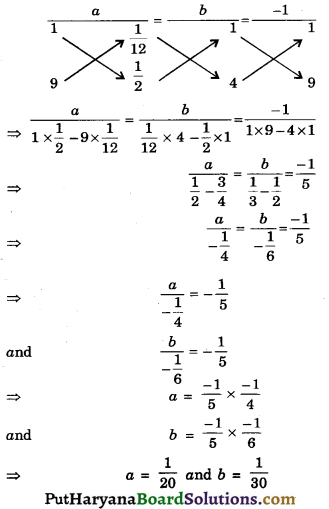Putting the values of a and b, we get
$$\frac{1}{x}=\frac{1}{20}$$ and $$\frac{1}{y}=\frac{1}{30}$$
⇒ x = 20 and y = 30
Hence, time taken by larger pipe is 20 hours and smaller pipe is 30 hours.

Question 10.
Find the value of k for which are roots of the equation 3x2 – 10x + k = 0 are reciprocal of each other.
Solution :
The given equation is :
3x2 – 10x + k = 0
Let one root of the equation be a since roots are reciprocal each other so, other root is $$\frac {1}{α}$$
Product of two roots = $$\frac {c}{a}$$
⇒ α × $$\frac {1}{α}$$ = $$\frac {k}{3}$$
⇒ 1 = $$\frac {k}{3}$$
⇒ k = 3Question 11.
For what value of k, the given quadratic equation kx2 – 6x – 1 = 0 has no real roots ?
Solution :
The given equation is :
kx2 – 6x – 1 = 0
The condition for no real roots is :
D < 0
⇒ b2 – 4ac < 0
⇒ (-6)2 – 4 × k × -1 < 0
⇒ 36 + 4k < 0
⇒ 4k < – 36
⇒ k < –$$\frac {36}{4}$$
⇒ k < – 9
Hence, k should be less than – 9 i.e., (-10, – 11, …..).

Question 12.
For what values of k, the roots of the equation kx2 + 4x + k = 0 are real ?
Solution :
The given equation is :
x2 + 4x + k = 0
∵ The given equation has real roots
∴ D ≥ 0
⇒ b2 – 4ac ≥ 0
⇒ (4)2 – 4 × 1 × k ≥ 0
⇒ 16 – 4k ≥ 0
⇒ – 4k ≥ – 16
⇒ k ≤ $$\frac {-16}{-4}$$
⇒ k ≤ + 4
Therefore, k should be ≤ 4 i.e., (4, 3, 2, 1, ….)Question 13.
Find the k so that the quadratie equation (k + 1)x2 – 2 (k + 1)x + 1 = 0 has equal roots.
Solution :
The given equation is :
(k + 1)x2 – 2(k + 1) x + 1 = 0
Since, equation has equal roots
∴ D = 0
⇒ b2 – 4ac = 0
⇒ [-2(k + 1)]2 – 4 × (k + 1) × 1 = 0
⇒ 4(k2 + 2k + 1) – 4k – 4 = 0
⇒ 4k2 + 8k + 4 – 4k – 4 = 0
⇒ 4k2 + 4k = 0
⇒ 4k(k + 1) = 0 and k + 1 = 9
⇒ k = – 1
k = – 1 (Reject since if we put the k = – 1 in the equation (-1 + 1)x will be zero
k = 0

Question 14.
If – 3 is a root of the quadratic equation 2x2 + Px – 15 = 0 while the quadratic equation x2 – 4Px + k = 0 has equal root. Find the value of k.
Solution :
Since, – 3 is a root of the equation
2x2 + Px – 15 = 0.
So, we put x = – 3 in this equation, we get
2(-3)2 + P(-3) – 15 = 0
⇒ 18 – 3P – 15 = 0
⇒ 3 – 3P = 0
⇒ P = $$\frac {-3}{-3}$$ = 1
Putting the value of P in the equation, we get
x2 – 4 × 1 + k = 0
⇒ x2 – 4x + k = 0
The equation x2 – 4x + k = 0 has equal roots.
So, D = 0
⇒ b2 – 4ac = 0
⇒ (-4)2 – 4 × 1 × k = 0
⇒ 16 – 4k = 0
⇒ k = $$\frac {-16}{-4}$$
⇒ k = 4

Question 15.
If the roots of the quadratic equation (x – a) (x – b) + (x – b) (x – c) + (x – c)(x – a) = 0 are equal, then show that a = b = c.
Solution :
The given equation is :
(x – a) (x – b) + (x – b) (x – c) + (x – c)(x – a) = 0
x2 – ax – bx + ab + x2 – bx – xc + bc + x2 – cx – ax + ac = 0
⇒ 3x2 – 2ax – 2x – 2x + ab + bc + ac = 0
⇒ 3x2 – 2 (a + b + c) x + (ab + bc + ac) = 0
Since, roots are equal, then
D = 0
⇒ b2 – 4ac = 0
⇒ [- 2 (a + b + c)]2 – 4 × 3 × (ab + bc + ac) = 0
⇒ 4(a2 + b2 + c2 + 2ab + 2bc + 2ca) – 12 (ab + bc + ac) = 0
⇒ 4[a2 + b2 + c2 + 2ab + 2bc + 2ca – 3ab – 3bc – 3ac] = 0
⇒ a2 + b2 + c2 – ab – bc – ac = 0
⇒ $$\frac {1}{2}$$[2a2 + 2b2 + 2c2 – 2ab – 2bc – 2ac] = 0
⇒ $$\frac {1}{2}$$[(a2 + b2 – 2ab) + (b2 + c2 – 2bc) + (a2 + c2 – 2ac)] = 0
⇒ (a – b)2 + (b – c)2 + (a – c)2 = 0
⇒ (a – b)2 = 0, (b – c)2 = 0, (a – c)2 = 0
⇒ a = b, b = c, a = c
∴ a = b = c
Hence Proved.Question 16.
If the root of the quadratic equation (c2 – ab)x2 – 2 (a2 – bc)x + b2 – ac = 0 in x are equal, then show that either a = 0 or a3 + b3 + c3 = 3abc.
Solution :
The given equation is:
(c2 – ab)x2 – 2(a2 – bc)x + (b2 – ac) = 0
Since, roots are equal, then
D = 0
⇒ b2 – 4ac = 0
⇒ [- 2(a2 – bc)]2 – 4 × (c2 – ab) (b2 – ac) = 0
⇒ 4(a4 + b2c2 – 2abc) – 4(b2c2 – ac3 – ab3 + a2bc) = 0
⇒ 4[a4 + b2c2 – 2a2bc – b2c2 + ac3 + ab3 – a2bc) = 0
⇒ [a4 – 3a2bc + ac3 + ab3] = 0
⇒ a[a3 + b3 + c3 – 3abc] = 0
⇒ a = 0 or a3 + b3 + c3 – 3abc = 0
⇒ a = 0 or a3 + b3 + c3 = 3abc
Hence proved.

Question 17.
A pole has to erected at a point on the boundary of a circular park of diameter 17 metres in such a way that the differences of its distances from two dimetrically opposite fixed gates A and B on the boundary is 7 metres. It is possible to do so? If yes, at what distances from the two gates should the pole be erected ?
Solution :
Let us first draw the diagram. Let P be location of the pole. Let the distance of pole from the gate B be x m ie., BP = x m. Now distances of the pole from the two gates = AP – BP (or BP – AP) = 7 m.
Therefore AP = (x + 7) m.
Now,
AB = 17 m.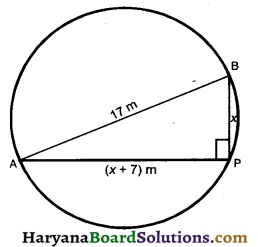∠APB = 90° (angle in a pamicircle is 90°) In a right triangle APB, we have
AB2 = AP2 + BP2
[By Pythagoaes theorem]
⇒ 172 = (x + 7)2 + x2
⇒ 289 = x2 + 49 + 14x + x2
⇒ 289 = 2x2 + 14x + 49
⇒ 2x2 + 14x + 49 – 289 = 0
⇒ x2 + 7x – 120 = 0
⇒ x2 + (15 – 8) x – 120 = 0
⇒ x2 + 15x – 8x – 120 = 0
⇒ x(x + 15) – 8(x + 15) = 0
⇒ (x – 8) (x + 15) = 0
⇒ x – 8 = 0 or x + 15 = 0
⇒ x = 8 or x = – 15 (Reject)
Hence, the pole has to be erected on the boundary of the park at a distance of 8 m from gate Band 8 + 7 i.e., 15 m from gate A.

Fill in the Blanks

Question 1.
A quardratic equation ax2 + bx + c = 0 has two………..real roots, if b2 – 4ac > 0.
Solution :
DistinctQuestion 2.
A quadratic equation ax2 + bx + c = 0 has no ………… roots, if b2 – 4ac < 0.
Solution :
Real

Question 3.
If we can factorise ax2 + bx + c, a ≠ 0, into a product of two linear factors, then the roots of the quadratic equation ax2 + bx + c = 0 can be found by equating each ………. to zero.
Solution :
Factor

Question 4.
A quadratic equation can also be solved by the method of completing the …………..
Solution :
Square

Question 5.
ar2 + bx + c = 0, a ≠ 0 is called the standard form in a quadratic ………..
Solution :
EquationQuestion 6.
A quadratic equation has atmost ……….. roots.
Solution :
Two.

Multiple Choice Questions

Question 1.
The root of the quadratic equation x2 – 0.04 = 0 are:
(a) ± 0.2
(b) ± 0.02
(c) 0.4
(d) 2
Solution :
(a) ± 0.2

x2 – 0.04 = 0
⇒ x2 – (0.2)2 = 0
⇒ (x + 0.2) (x – 0.2) = 0
⇒ x + 0.2 = 0
and x – 0.2 = 0
⇒ x = – 0.2
and x = 0.2
⇒ x = ± 0.2Question 2.
If x2 + 2kx + 4 = 0 has a root x = 2, then value of k is:
(a) – 1
(b) – 2
(c) 2
(d) – 4.
Solution :
(b) – 2

x2 + 2kx + 4 = 0
one root x = 2 then
(2)2 + 2k(2) + 4 = 0
4k + 8 = 0
⇒ k = – 2
So correct choice is (b).

Question 3.
(x2 + 1)2 – x2 = 0 has
[NCERT Exemplar Problems]
(a) four real roots
(b) two real roots
(c) no real roots
(d) one real roots.
Solution :
(c) no real roots

(x2 + 1)2 – x2 = 0 get x2 = y, then
(y + 1)2 – y = 0
y2 + 1 + 2y – y = 0
y2 + y + 1 = 0
∵ b2 < 4ac
So, no real root.
Hence correct choice is (c).

Question 4.
Which of the following equations has two distinct real roots ?
[NCERT Exemplar Problems]
(a) 2x2 – 3$$\sqrt{2}$$x + $$\frac {9}{4}$$
(b) x2 + x – 5 = 0
(c) x2 + 3x + 2$$\sqrt{2}$$ = 0
(d) 5x2 – 3x + 1 = 0.
Solution :
(b) x2 + x – 5 = 0

(a) 2x2 – 3$$\sqrt{2}$$x + $$\frac {9}{4}$$ = 0
D = (-3$$\sqrt{2}$$)2 – 4 × 2 × $$\frac {9}{4}$$ = 0

(b) x2 + x – 5 = 0
D = (1)2 – (4) (1) (-5) = 21
D > 1
Hence correct choice is (b).Question 5.
The roots of the equation 3x2 – 4x + 3 = 0 are :
(a) real and unequal
(b) real and equal
(c) imaginary
(d) none of these.
Solution :
(c) imaginary

3x2 – 4x + 3 = 0
a = 3, b = – 4, c = 3
b2 = (-4)2 = 16
4ac = 4 × 3 × 3 = 36
∵ b2 < 4ac
So roots are imaginary Hence correct choice (c).